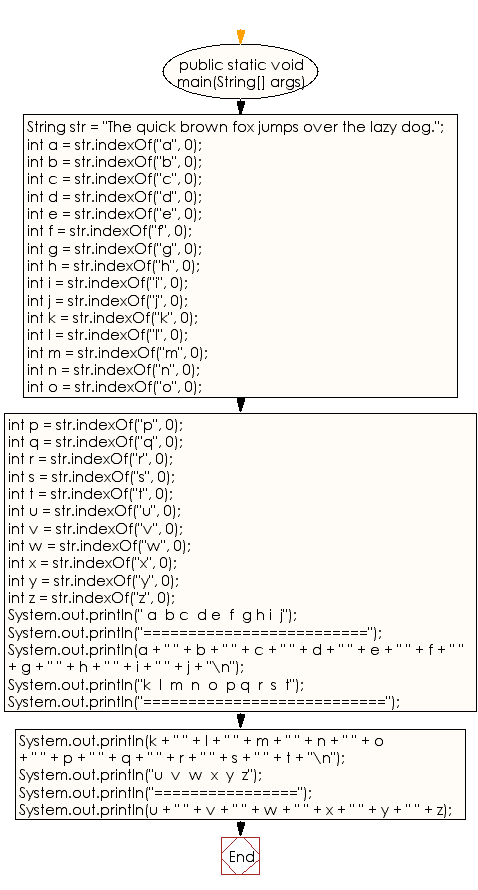﻿ Java - Get the index of all the characters of the alphabet

# Java: Get the index of all the characters of the alphabet

## Java String: Exercise-19 with Solution

Write a Java program to get the index of all the characters of the alphabet.

Sample string of all alphabet: "The quick brown fox jumps over the lazy dog."

Sample Solution:

Java Code:

``````// Define a public class named Exercise19.
public class Exercise19 {
// Define the main method.
public static void main(String[] args) {
// Declare and initialize a string variable.
String str = "The quick brown fox jumps over the lazy dog.";

// Get the index of all the characters of the alphabet
// starting from the beginning of the String.
int a = str.indexOf("a", 0);
int b = str.indexOf("b", 0);
int c = str.indexOf("c", 0);
int d = str.indexOf("d", 0);
int e = str.indexOf("e", 0);
int f = str.indexOf("f", 0);
int g = str.indexOf("g", 0);
int h = str.indexOf("h", 0);
int i = str.indexOf("i", 0);
int j = str.indexOf("j", 0);
int k = str.indexOf("k", 0);
int l = str.indexOf("l", 0);
int m = str.indexOf("m", 0);
int n = str.indexOf("n", 0);
int o = str.indexOf("o", 0);
int p = str.indexOf("p", 0);
int q = str.indexOf("q", 0);
int r = str.indexOf("r", 0);
int s = str.indexOf("s", 0);
int t = str.indexOf("t", 0);
int u = str.indexOf("u", 0);
int v = str.indexOf("v", 0);
int w = str.indexOf("w", 0);
int x = str.indexOf("x", 0);
int y = str.indexOf("y", 0);
int z = str.indexOf("z", 0);

// Display the results of all the indexOf method calls.
System.out.println(" a  b c  d e  f  g h i  j");
System.out.println("=========================");
System.out.println(a + " " + b + " " + c + " " + d + " " +
e + " " + f + " " + g + " " + h + " " +
i + " " + j + "\n");

System.out.println("k  l  m  n  o  p q  r  s  t");
System.out.println("===========================");
System.out.println(k + " " + l + " " + m + " " + n + " " +
o + " " + p + " " + q + " " + r + " " +
s + " " + t + "\n");

System.out.println("u  v  w  x  y  z");
System.out.println("================");
System.out.println(u + " " + v + " " + w + " " + x + " " +
y + " " + z);
}
}
```
```

Sample Output:

```a  b c  d e  f  g h i  j
=========================
36 10 7 40 2 16 42 1 6 20

k  l  m  n  o  p q  r  s  t
===========================
8 35 22 14 12 23 4 11 24 31

u  v  w  x  y  z
================
5 27 13 18 38 37
```

Flowchart:Java Code Editor:

Improve this sample solution and post your code through Disqus

What is the difficulty level of this exercise?

Test your Programming skills with w3resource's quiz.

﻿